# Technique

## Assigning rank order to the instances of a population submode

ModelId:
ranking1
SimileVersion:
3.1

There are a number of situations where you might want to rank the members (instances) of a population submodel in order, according to some criterion.

Category:
Technique
Equations:

Equations in submodel 'individual'

attribute = rand_const(0,100)
rank = count({one_role2})+1
where: {one_role1} = ../ranking/one (to individual in role1)
creation: initial number = 10

Equations in submodel 'ranking'
condition = (attribute_role1>attribute_role2)
where:
attribute_role1 = ../individual/attribute (from individual in role1)
attribute_role2 = ../individual/attribute (from individual in role2)]
one = 1

Model tags:

## Skeleton cohort model

ModelId:
cohort1
SimileVersion:
5.97

This example shows how to implement a basic cohort model in Simile.

A cohort model is one way of modelling a population. The individuals are grouped into cohorts, typically on the basis that they were born at the same time. Each cohort has as a minimum a variable representing the number of individuals in that cohort, along with possibly other cohort-specific attributes, such as the average size of the individuals in the cohort.

Equations:

Variable: n_total = sum({n})

Where: {n} = Cohort/n

Variable: population_total_size = sum({cohort_total_size})
Where: {cohort_total_size} = Cohort/cohort_total_size

--------------------------------------------------------------
Submodel Bigger

Submodel "Bigger" is an association submodel between "Cohort" and itself with roles "other" and "me".

Condition: condition = other_size>me_size
Where:
me_size = Value(s) of ../Cohort/size from submodel "Cohort" in role "me"
other_size = Value(s) of ../Cohort/size from submodel "Cohort" in role "other"

Variable: total_size_of_bigger_cohort = other_cohort_total_size
Where:
me_cohort_total_size = Value(s) of ../Cohort/cohort_total_size from submodel "Cohort" in role "me"
other_cohort_total_size = Value(s) of ../Cohort/cohort_total_size from submodel "Cohort" in role "other"

-------------------------------------------------------------------------
Submodel Cohort

Submodel "Cohort" is a population submodel.

Compartment: n
Initial value = 100
Rate of change = - loss

Compartment: size
Initial value = 0
Rate of change = + growth

Flow: growth = gr*(1-size/max_size)

Flow: loss = 5

Immigration: create_new_cohort = 1

Loss: remove_cohort = n<=0

Variable: cohort_total_size = n*size

Variable: gr = 1.5

Variable: max_size = 10

Variable: total_size_of_all_bigger_cohorts = sum({cohort_total_size_of_bigger_me})
Where:
{cohort_total_size_of_bigger_me} = ../Bigger/total_size_of_bigger_cohort for submodel "Cohort" in role "me"

Results:

The results shown here illustrate the basic functioning of this model.

The following figure shows a set of panels, each one showing the values for a specified variable at time 10. These were made using Simile's 'snapshot' tool, and enable us to trace the values as they are processed in the model.The first 3 panels on the top row show, for each of the 10 cohorts created up to this time, the number of tress (n) and the size of the average tree (size) in the cohort, along with the total size (n x size). You can see that the number of tress declines in a cohort declines by 5 each time step, and that the average tree in a cohort grows at a reducing rate as the cohort gets older.

The bottom window shows the total_size values for the individual cohorts that are bigger than the specified cohort. Thus, cohort 2 only has one value (since there is only one bigger than it is), while cohort 3 has 2 values, and so on.

The top-right window shows 'total_size_of_all_bigger_cohorts' - the sum (along a row) for al the total_size values of bigger cohorts for a particular cohort. This is the key value which enables the impact of bigger cohorts on the growth of a cohort to be modelled.

The graph below shows how the 'total_size_of_all_bigger_cohorts' changes over time, for each cohort. The lowest curve is for the second cohort (since the first cohort has nothing bigger than it. Each successive cohort starts off at a higher value (since the total size of cohorts above it is increasing), until we get to the point where each newly-created cohort starts off with the same total_size above it, which decreases as that cohort in turn increases in size.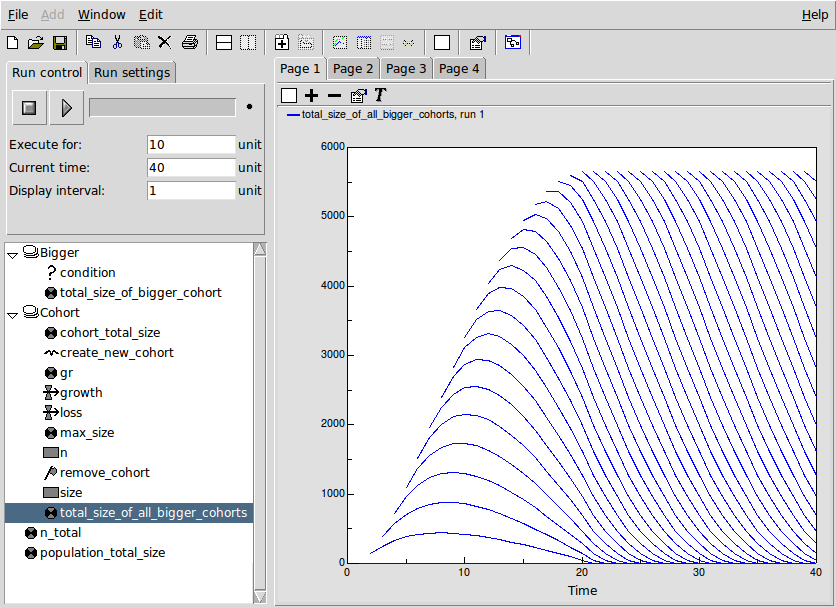## Iterative solution to Ball-Berry stomatal conductance simultaneous equations (Simile V4+)

ModelId:
BallBerry4a
SimileVersion:
5.9

The Ball-Berry stomatal conductance poses a problem for conventional System Dynamics modelling tools because it depends upon the solution of the following pair of simultaneous equations.

Gs = g0 + g1 * A * H / Ca
A = Gs * AQ

Equations:

Equations in BallBerry4aP

Equations in Environment

Variable C_a : Carbon dioxide concentration (umol CO2 (mol air)^-1)
C_a = graph(time())
Typical diurnal curve in forest canopy

Variable H : Relative humidity (proportion)
H = graph(time())
Typical diurnal graph (24 hour)

Variable Q : Photon flux density (umol m^-2 s^-1)
Q = graph(time())
Graph for a sunny day (24 hours)

Equations in Ball-Berry

Variable Gs_start
Gs_start = if time()==0 then g_0 else last(Gs_0)
Where:
Gs_0=Iteration time step/Gs_0

Variable g_0 : Stomatal conductance in the dark (mol m^-2 s^-1)
g_0 = 0.01

Variable g_1 : Ball-Berry stomatal conductance coefficient
g_1 = 23

Equations in Iteration time step

Alarm
Variable Gs
Gs = if loop_count==0 then Gs_start else Gs_0
Where:
Gs_start=../Gs_start

Variable Gs_0 : Stomatal conductance (mol m^-2 s^-1)
Gs_0 = g_0+g_1*A*H/C_a
Where:
A=Assimilation/A
H=../../Environment/H
C_a=../../Environment/C_a
g_0=../g_0
g_1=../g_1
Ball-Berry equation

Variable loop_count
loop_count = iterations(al1)

Equations in Assimilation

Variable A : Assimation (umol CO2 m^-2 s^-1)
A = A_Q*Gs
Where:
Gs=../Gs

Variable A_Q : Assimilation light response curve
A_Q = graph(Q)
Where:
Q=../../../Environment/Q
Relationship of Assimilation with photon flux density (light) when stomatal conductance (Gs) is maximum

Results: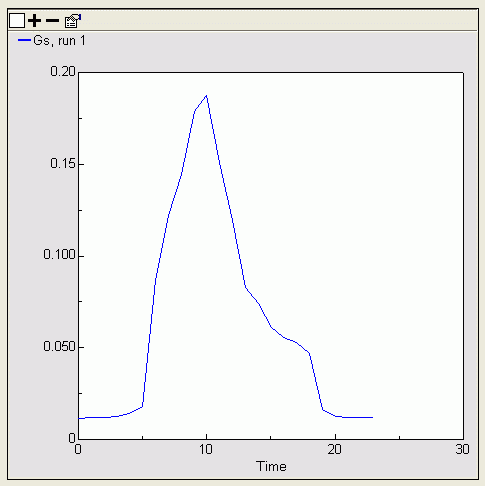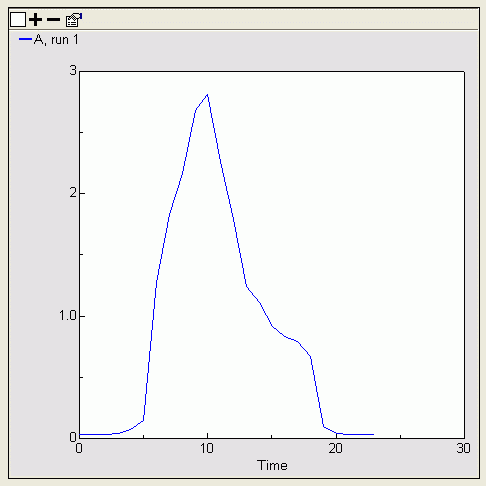Plot of stomatal conductance versus time            Plot of assimilation versus time

## Iterative solution to Ball-Berry stomatal conductance simultaneous equations (Simile V3+)

ModelId:
ballberry1
SimileVersion:
3.1+

Here’s an example of a model with two simultaneous equations implemented in Simile. It is a version of the Ball-Berry stomatal conductance model where assimilation (photosynthesis) is a function of stomatal conductance and stomatal conductance is a function of assimilation.

Where there is a big change in stomatal conductance (Gs) more iterations are needed to reach a certain accuracy.

Category:
Specific
Equations:

Equations in Desktop

Equations in Assimilation

Variable A : Assimation (umol CO2 m^-2 s^-1)
A = A_Q*last(Gs_0)
Where:
Gs_0=../Gs

Variable A_Q : Assimilation light response curve
A_Q = graph(Q)
Where:
Q=../../../Environment/Q
Relationship of Assimilation with photon flux density (light) when stomatal conductance (Gs) is maximum

Equations in Environment

Variable C_a : Carbon dioxide concentration (umol CO2 (mol air)^-1)
C_a = graph(time())
Typical diurnal curve in forest canopy

Variable H : Relative humidity (proportion)
H = graph(time())
Typical diurnal graph (24 hour)

Variable Q : Photon flux density (umol m^-2 s^-1)
Q = graph(time())
Graph for a sunny day (24 hours)

Equations in Iteration

Variable Gs : Stomatal conductance (mol m^-2 s^-1)
Gs = if time()==time then Gs else g_0+g_1*A*H/C_a
Where:
A=Assimilation/A
time=../time
H=../../Environment/H
C_a=../../Environment/C_a
g_0=../g_0
g_1=../g_1
Gs=../Gs
Ball-Berry equation

Variable errorGs
errorGs = Gs_0-last(Gs_0)
Where:
Gs_0=Gs

Equations in Ball-Berry

Variable A
A = A
Where:
A=Iteration/Assimilation/A

Variable Gs
Gs = if time()==0 then g_0 else last(Gs_0)
Where:
Gs_0=Iteration/Gs

Variable errorGs
errorGs = last(errorGs_0)
Where:
errorGs_0=Iteration/errorGs

Variable g_0 : Stomatal conductance in the dark (mol m^-2 s^-1)
g_0 = 0.01

Variable g_1 : Ball-Berry stomatal conductance coefficient
g_1 = 23

Variable time
time = time()

Results:

This first pair of graphs shows the calculated Stomatal Conductance and Assimilation on an hour-by-hour basis throughout the day.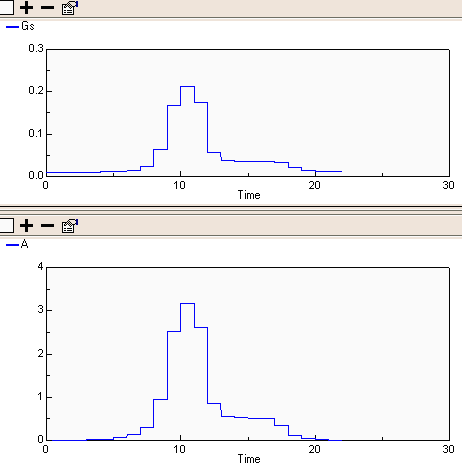This second pair shows the internal model representation of the two variables converging on the required hourly value. Note that when the magnitude of changes in the variables is large, as it is around time()==10 units, the convergence takes longer.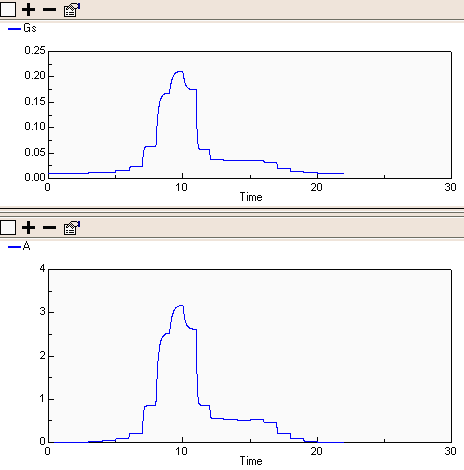References:

J. T. Ball, I. E. Woodrow, J. A. Berry. 1987. A model predicting stomatal conductance and its contribution to the control of photosynthesis under different environmental conditions. In: Progress in Photosynthesis Research, ed. J. Biggins. M. Nijhoff Publishers, Dordrecht. Vol. 4, pp 221-224.